# GEMSEO in 10 minutes¶

## Introduction¶

This is a short introduction to GEMSEO, geared mainly for new users. In this example, we will set up a simple Multi-disciplinary Design Optimization (MDO) problem based on a simple analytic problem.

## Imports¶

First, we will import all the classes and functions needed for the tutorials. The first imports (__future__ and future) enable to run the tutorial using either a Python 2 or a Python 3 interpreter.

from __future__ import absolute_import, division, unicode_literals

from math import exp, sqrt

from future import standard_library
from numpy import array, ones


Finally, the following functions from the GEMSEO API are imported. They will be used latter in order to instantiate GEMSEO objects.

from gemseo.api import (
configure_logger,
create_design_space,
create_discipline,
create_scenario,
)

configure_logger()


Out:

<RootLogger root (INFO)>


These imports enables to compute mathematical expressions, as well to instantiate numpy arrays. Numpy arrays are used to store numerical data in GEMSEO at low level. If you are not confortable with using Numpy, please have a look at the Numpy Quickstart tutorial.

standard_library.install_aliases()


## A simple MDO test case: the Sellar Problem¶

We will consider in this example the Sellar’s problem:

\begin{split}\begin{aligned} \text{minimize the objective function }&obj=x_{local}^2 + x_{shared,1} +y_0+e^{-y_1} \\ \text{with respect to the design variables }&x_{shared},\,x_{local} \\ \text{subject to the general constraints } & c_1 \leq 0\\ & c_2 \leq 0\\ \text{subject to the bound constraints } & -10 \leq x_{shared,0} \leq 10\\ & 0 \leq x_{shared,1} \leq 10\\ & 0 \leq x_{local} \leq 10. \end{aligned}\end{split}

where the coupling variables are

$\text{Discipline 0: } y_0 = x_{shared,0}^2 + x_{shared,1} + x_{local} - 0.2\,y_1,$

and

$\text{Discipline 1: }y_1 = \sqrt{y_0} + x_{shared,0} + x_{shared,1}.$

and where the general constraints are

\begin{align}\begin{aligned}c_0 = 1-y_0/{3.16}\\c_1 = y_1/{24.} - 1\end{aligned}\end{align}

## Definition of the disciplines using Python functions¶

The Sellar’s problem is composed of two disciplines and an objective function. As they are expressed analytically, it is possible to write them as simple Python functions which take as parameters the design variables and the coupling variables. The returned values may be the outputs of a discipline, the values of the constraints or the value of the objective function. Their definitions read:

def f_sellar_system(x_local=1.0, x_shared_1=3.0, y_0=1.0, y_1=1.0):
"""
Objective function
"""
obj = x_local ** 2 + x_shared_1 + y_0 + exp(-y_1)
c_0 = 1 - y_0 / 3.16
c_1 = y_1 / 24.0 - 1.0
return obj, c_0, c_1

def f_sellar_0(x_local=1.0, y_1=1.0, x_shared_0=1.0, x_shared_1=3.0):
"""
Function for discipline 0
"""
y_0 = x_shared_0 ** 2 + x_shared_1 + x_local - 0.2 * y_1
return y_0

def f_sellar_1(y_0=1.0, x_shared_0=1.0, x_shared_1=3.0):
"""
Function for discipline 1
"""
y_1 = sqrt(y_0) + x_shared_0 + x_shared_1
return y_1


These Python functions can be easily converted into GEMSEO MDODiscipline objects by using the AutoPyDiscipline discipline. It enables to automatically wrap a Python function into a GEMSEO MDODiscipline by only passing a reference to the function to be wrapped. GEMSEO handles the wrapping and the grammar creation under the hood. The AutoPyDiscipline discipline can be instanciated using the create_discipline() function from the GEMSEO API:

disc_sellar_system = create_discipline("AutoPyDiscipline", py_func=f_sellar_system)

disc_sellar_0 = create_discipline("AutoPyDiscipline", py_func=f_sellar_0)

disc_sellar_1 = create_discipline("AutoPyDiscipline", py_func=f_sellar_1)


Note that it is possible to define the Sellar disciplines by subclassing the MDODiscipline class and implementing the constuctor and the _run method by hand. Although it would take more time, it may also provide more flexibily and more options. This method is illustrated in the Sellar from scratch tutorial.

# We then create a list of disciplines, which will be used later to create a
# :class:.MDOScenario:
disciplines = [disc_sellar_system, disc_sellar_0, disc_sellar_1]


Note

For the sake of clarity, these disciplines are overly simple. Yet, GEMSEO enables to define much more complex disciplines, such as wrapping complex COTS. Check out the other tutorials and our publications list for more information.

## Definition of the design space¶

In order to define MDOScenario, a design space has to be defined by creating a DesignSpace object. The design space definition reads:

design_space = create_design_space()


## Definition of the MDO scenario¶

Once the disciplines and the design space have been defined, we can create our MDO scenario by using the create_scenario() API call. In this simple example, we are using a Multiple Disciplinary Feasible (MDF) strategy. The Multiple Disciplinary Analyses (MDA) are carried out using the Gauss-Seidel method. The scenario definition reads:

scenario = create_scenario(
disciplines,
formulation="MDF",
main_mda_class="MDAGaussSeidel",
objective_name="obj",
design_space=design_space,
)


It can be noted that neither a workflow nor a dataflow has been defined. By design, there is no need to explicitely define the workflow and the dataflow in GEMSEO:

• the workflow is determined from the MDO formulation used.

• the dataflow is determined from the variable names used in the disciplines. Then, it is of utermost importance to be consistant while choosing and using the variable names in the disciplines.

Warning

As the workflow and the dataflow are implicitely determined by GEMSEO, set-up errors may easily occur. Although it is not performed in this example, it is strongly advised to

• check the interfaces between the several disciplines using a N2 diagram,

• check the MDO process using an XDSM representation

## Setting the constraints¶

Most of the MDO problems are under constraints. In our problem, we have two inequality constraints, and their declaration reads:

scenario.add_constraint("c_0", "ineq")


## Execution of the scenario¶

The scenario is now complete and ready to be executed. When running the optimization process, the user can choose the optimization algorithm and the maximum number of iterations to perform. The execution of the scenario reads:

scenario.execute(input_data={"max_iter": 10, "algo": "SLSQP"})


Out:

{'max_iter': 10, 'algo': 'SLSQP'}


The scenario is converged after 7 iterations. Useful information can be found in the standard output, as shown below:

*** Start MDO Scenario execution ***
MDOScenario:
Disciplines: f_sellar_system f_sellar_0 f_sellar_1
MDOFormulation: MDF
Algorithm: SLSQP

Optimization problem:
Minimize: obj(x_local, x_shared_0, x_shared_1)
With respect to:
x_local, x_shared_0, x_shared_1
Subject to constraints:
c_0(x_local, x_shared_0, x_shared_1) <= 0
c_1(x_local, x_shared_0, x_shared_1) <= 0
Design Space:
+------------+-------------+-------+-------------+-------+
| name       | lower_bound | value | upper_bound | type  |
+------------+-------------+-------+-------------+-------+
| x_local    |      0      |   1   |      10     | float |
| x_shared_0 |     -10     |   4   |      10     | float |
| x_shared_1 |      0      |   3   |      10     | float |
+------------+-------------+-------+-------------+-------+
Optimization: |          | 0/10   0% [elapsed: 00:00 left: ?, ? iters/sec]
Optimization: |██████    | 6/10  60% [elapsed: 00:00 left: 00:00, 56.08
iters/sec obj:  3.18 ]
Optimization result:
Objective value = 3.18339398657
The result is feasible.
Status: 0
Optimizer message: Optimization terminated successfully.
Number of calls to the objective function by the optimizer: 7
Constraints values:
c_1 = -0.843530155261
c_0 = 1.59205981731e-13

Design Space:
+------------+-------------+-----------------------+-------------+-------+
| name       | lower_bound |         value         | upper_bound | type  |
+------------+-------------+-----------------------+-------------+-------+
| x_local    |      0      |           0           |      10     | float |
| x_shared_0 |     -10     |    1.97763739026546   |      10     | float |
| x_shared_1 |      0      | 5.698379135171542e-13 |      10     | float |
+------------+-------------+-----------------------+-------------+-------+
*** MDO Scenario run terminated in 0:00:00.118049 ***


Note

GEMSEO provides the user with a lot of optimization algorithms and options. An exhaustive list of the algorithms available in GEMSEO can be found in the Optimization algorithms options section.

## Post-processing the results¶

Post-processings such as plots exhibiting the evolutions of the objective function, the design variables or the constraints can be extremely useful. The convergence of the objective function, design variables and of the inequality constraints can be observed in the following plots. Many other post-processings are available in GEMSEO and are described in Post-processing.

scenario.post_process("OptHistoryView", save=False, show=True)

•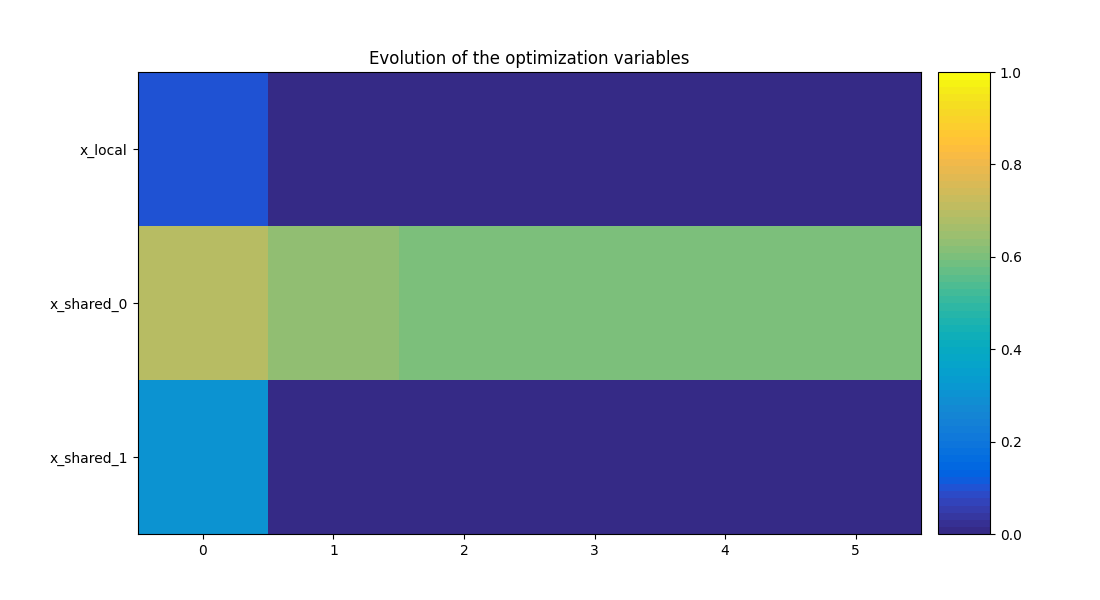•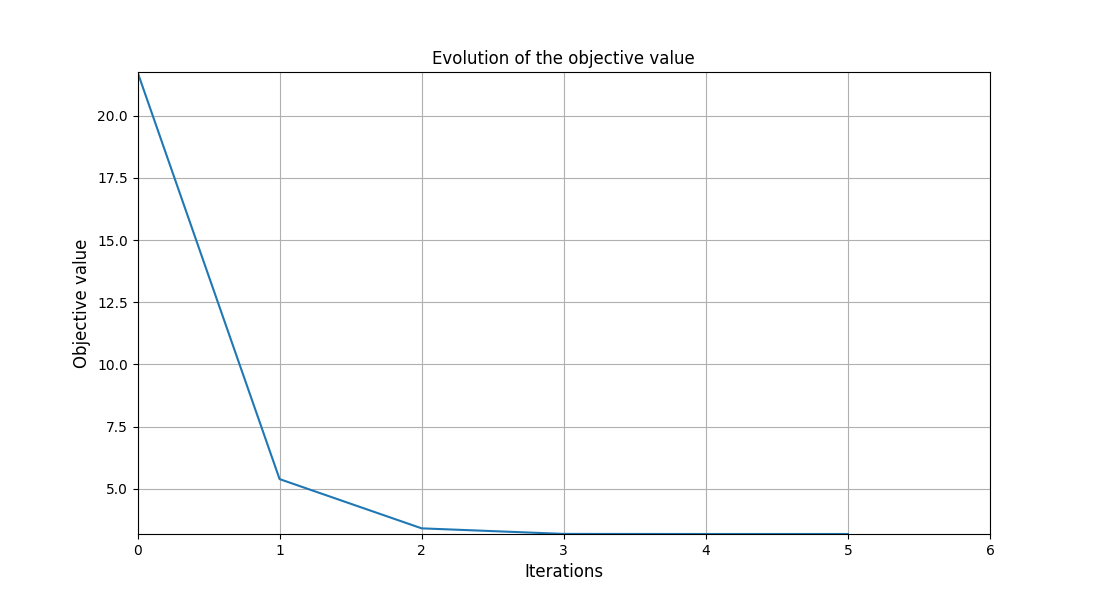•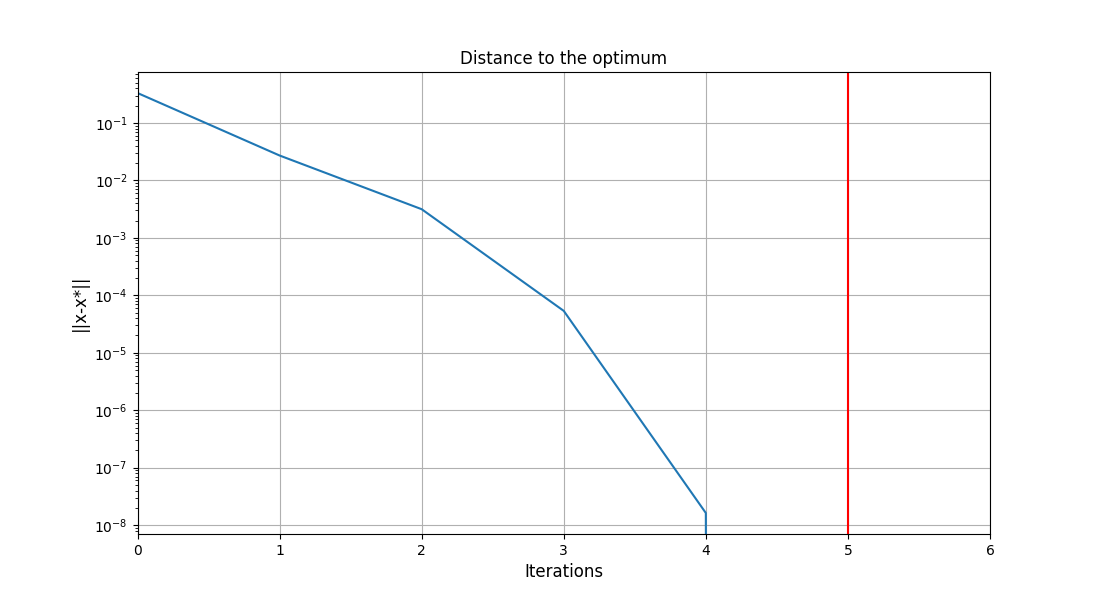•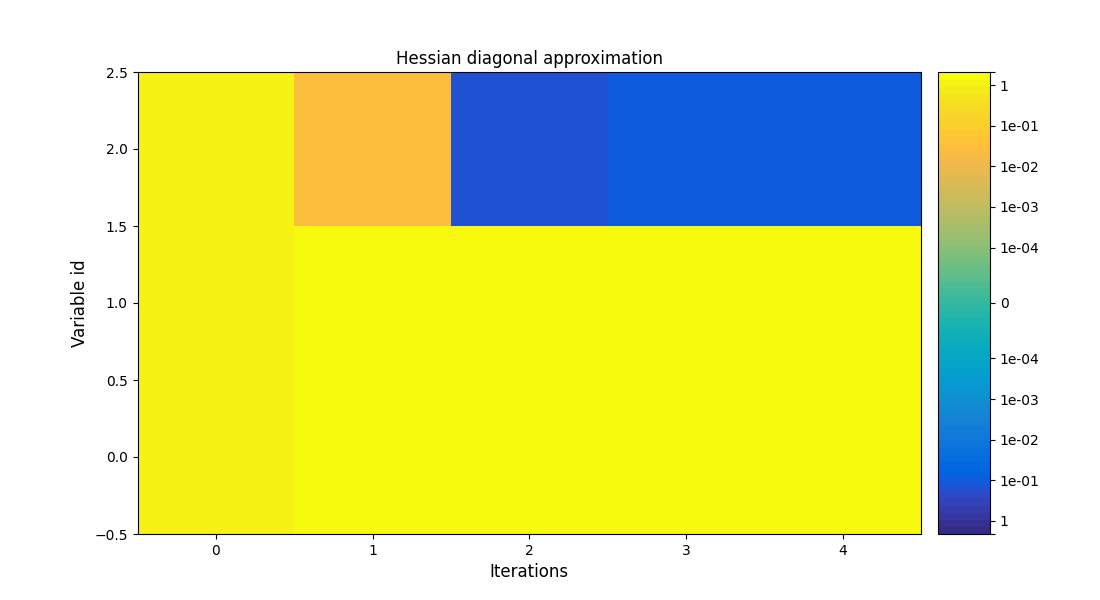•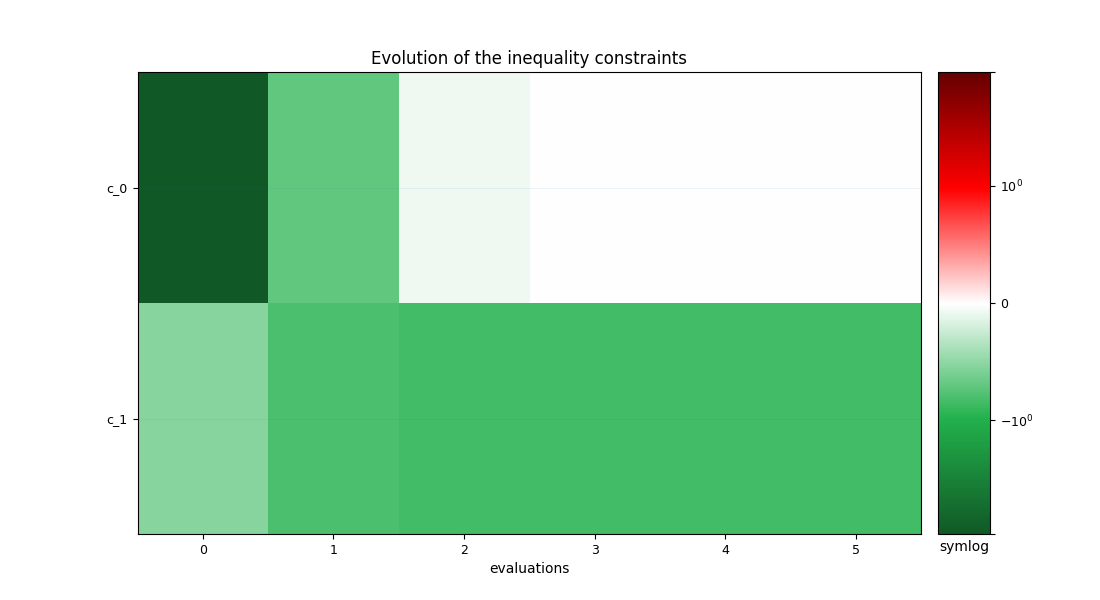Out:

/home/docs/checkouts/readthedocs.org/user_builds/gemseo/conda/3.0.3/lib/python3.8/site-packages/gemseo/post/opt_history_view.py:312: UserWarning: FixedFormatter should only be used together with FixedLocator
ax1.set_yticklabels(y_labels)
/home/docs/checkouts/readthedocs.org/user_builds/gemseo/conda/3.0.3/lib/python3.8/site-packages/gemseo/post/opt_history_view.py:716: MatplotlibDeprecationWarning: default base will change from np.e to 10 in 3.4.  To suppress this warning specify the base keyword argument.
norm=SymLogNorm(linthresh=linthresh, vmin=-vmax, vmax=vmax),
/home/docs/checkouts/readthedocs.org/user_builds/gemseo/conda/3.0.3/lib/python3.8/site-packages/gemseo/post/opt_history_view.py:626: MatplotlibDeprecationWarning: default base will change from np.e to 10 in 3.4.  To suppress this warning specify the base keyword argument.
norm=SymLogNorm(linthresh=1.0, vmin=-vmax, vmax=vmax),
/home/docs/checkouts/readthedocs.org/user_builds/gemseo/conda/3.0.3/lib/python3.8/site-packages/gemseo/post/opt_history_view.py:619: MatplotlibDeprecationWarning: Passing parameters norm and vmin/vmax simultaneously is deprecated since 3.3 and will become an error two minor releases later. Please pass vmin/vmax directly to the norm when creating it.
im1 = ax1.imshow(

<gemseo.post.opt_history_view.OptHistoryView object at 0x7fc299250580>


Note

Such post-processings can be exported in PDF format, by setting save to True and potentially additional settings (see the Scenario.post_process() options).

## What’s next?¶

You have completed a short introduction to GEMSEO. You can now look at the tutorials which exhibit more complex use-cases. You can also have a look at the documentation to discover the several features and options of GEMSEO.

Total running time of the script: ( 0 minutes 1.063 seconds)

Gallery generated by Sphinx-Gallery